# Whether the curve is the graph of a function of x .### Precalculus: Mathematics for Calcu...

6th Edition
Stewart + 5 others
Publisher: Cengage Learning
ISBN: 9780840068071### Precalculus: Mathematics for Calcu...

6th Edition
Stewart + 5 others
Publisher: Cengage Learning
ISBN: 9780840068071

#### Solutions

Chapter 2.2, Problem 51E

a.

To determine

## Whether the curve is the graph of a function of x .

Expert Solution

The curve of the graph is the function of x .

### Explanation of Solution

Given information :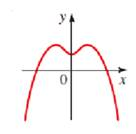Concept used : A curve in the coordinate plane is the graph of a function if and only if no vertical line intersects the curve more than once.No vertical line intersects the curve of the graph more than once, hence the curves of the graph is the function of x .

b.

To determine

### Whether the curve is the graph of a function of x .

Expert Solution

The curve of the graph is not the function of x .

### Explanation of Solution

Given information: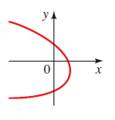Concept Used: A curve in the coordinate plane is the graph of a function if and only if no vertical line intersects the curve more than once.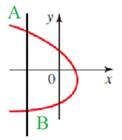Vertical line AB intersects the curve of graph twice. So, the curve of the graph is not a function of x .

c.

To determine

### Whether the curve is the graph of a function of x .

Expert Solution

The curve of the graph is function of x .

### Explanation of Solution

Given information: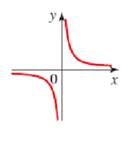Concept used : A curve in the coordinate plane is the graph of a function if and only if no vertical line intersects the curve more than once.

No vertical line intersects the curve of the graph more than once, hence the curves of the graph is the function of x .

d.

To determine

### Whether the curve is the graph of a function of x .

Expert Solution

The curve of the graph is not the function of x .

### Explanation of Solution

Given information :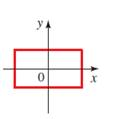Concept used : A curve in the coordinate plane is the graph of a function if and only if no vertical line intersects the curve more than once.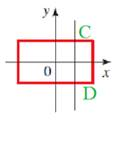Vertical line CD intersects the curve of graph twice. So, the curve of the graph is not a function of x .

### Have a homework question?

Subscribe to bartleby learn! Ask subject matter experts 30 homework questions each month. Plus, you’ll have access to millions of step-by-step textbook answers!